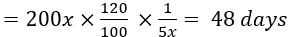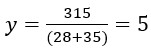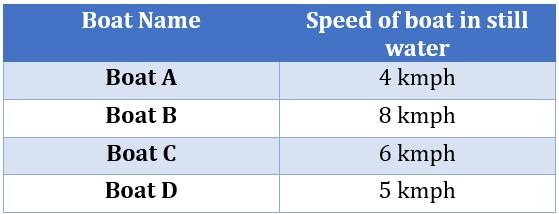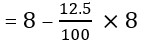# Arithmetic Data Interpretation – Part 1

## Arithmetic Data Interpretation – Part 1

Arithmetic Data Interpretation PDF for Banking Examination. This is helping in all upcoming baking examinations like SBI PO, IBPS PO, RBI Grade B, etc.

Direction (1 -5): The table given below shows the number of days taken by six people to complete a work D1. Read the data given in the table and answer the following questions.Note: Some data are missing, calculate if required.

Q1. If the ratio of efficiency of the Akash to Ajay is 5 : 8, then find the number of days taken by Akash to complete a work D2, which is 20% more than D1?
(a) 42 days
(b) 44 days
(c) 52 days
(d) 48 days
(e) 40 days

Ans (d). Let the work done by Ajay in one day = 8x units
And one day work of Akash = 5x units
Therefore, total work = 8x x 25 = 200x units
Required number of daysQ2. If Ram & Puneet working together complete the work D1 in ‘x’ days and Ajay & Rajeev working together complete the work D1 in y days, then choose the correct statement.
(a) x > y
(b) x < y
(c) x = y
(d) x + y > 30
(e) x + y < 20

Ans(b) Ram can complete the work in 20 days
punnet can complete the work in 45 days
Let total work = 180 (L.C.M of 20 and 45)
Efficiency (one day work) of Ram = 180/20 = 9 units
Efficiency (one day work) of Puneet = 180/45 = 4 units
Ram and punnet can complete the work  in x days = 180 / (9+4) = 180/13 = 13(11/13) days
Ajay can complete the work in 25 days
Rajeev can complete the work in 35 days
Let total work = 175 (L.C.M of 25 and 35)
Efficiency (one day work) of Ajay = 175/25 = 7 units
Efficiency (one day work) of Rajeev = 175/35 = 5 units
Ajay & Rajeev can complete the work in y days= 175 / (7+5) = 175/12 = 14(7/12) days
So, x < y

Q3. If efficiency of Deepak is 50% less than Ajay and both start working on alternate days starting with Deepak, then find the number of days requires to complete the work D1?
(a) 30 days
(b) 31(1/2) days
(c) 32 days
(d) 33(1/2) days
(e) 34 days

Ans.(d) Let the efficiency of Ajay is 2x units/day
Therefore efficiency of Deepak will beTotal work = 2x 25 = 50x units
Work done in 2 days =  x + 2x = 3x units
Work done in 32(2×16) days = 3x 16 = 48x units
Work done in 33 th day by Deepak  = x units
Remaining work = 50x – 48x – x = x units
Now the remaining work will be complete by Ajay in x/2x = 1/2 day
Total Number of days = 33(1/2) days

Q4. Ajay starts work D1 and after 5 days he left work. If both Ram and Puneet working together to complete the remaining work, then find number of days required to complete the remaining work?
(a) 10 days
(b) 11(1/13) days
(c) 12 (1/13) days
(d) 13 (7/13) days
(e) None of these

Ans.(b) Number of days taken by Ajay to complete the work = 25
Number of days taken by Ram to complete the work = 20
Number of days taken by Puneet to complete the work = 45
Let the total work = 900 units (lcm of 25, 20 and 45)
One day work of Ajay = 900/25 = 36 units
One day work of Ram = 900/20 = 45 units
One day work of Puneet = 900/45 = 20 units
Work completed by Ajay in 5 days = 36×5 = 180 units
Remaining work = 900 – 180 = 720 units
Remaining work complete by Ram and Puneet in
= 720 / (45+20) = 144/13 = 11(1/13) days

Q5. Ajay and Ram start working together to complete the work D1 but after ‘y’ days they both left the work and the remaining work is completed by Rajeev in 19(1/4) days. Find the value of y.
(a) 6.5
(b) 7
(c) 4
(d) 6
(e) 5

Ans (e) Number of days taken by Ajay to complete the work = 25 days
Number of days taken by Ram to complete the work = 20 days
Number of days taken by Rajeev to complete the work = 35 days
Let the total work = 700 (lcm of 25, 20 and 35)
One day work of Ajay = 700/25 = 28
One day work of Ram = 700/20 = 35
One day work of Rajeev = 700/35 = 20
Work done of Rajeev in 19(1/4) days = 20 x 19.25 = 385
Remaining work = 700 – 385 = 315 unitsDirections(6-10): The table given below shows speed of four different boats in still water. Read the data carefully and answer the questions.Q6. If the speed of the stream is 2 kmph, then find the total time taken by boat B and boat C to travel 16 km in upstream together?
(a) 6(2/3) hr
(b) 6(1/3) hr
(c) 7(2/3) hr
(d) 7(1/3) hr
(e) None of these

Ans(a) Upstream speed for boat B = 8 – 2 = 6 kmph
Time taken by boat B to travel 16 km upstream = 16/6 = 8/3 hr
Upstream speed for boat C = 6 – 2 = 4 kmph
Time taken by boat C to travel 16 km upstream = 16/4 = 4 hr
Total time = 4 + 8/3 = 20/3 = 6(2/3) hr

Q7. If speed of stream is 3 kmph, then find the ratio of distance covered in downstream by each boat D and boat C respectively?
(a) 1 : 4
(b) 3 : 5
(c) 4 : 3
(d) 2 : 3
(e) 4 : 5

Ans(e) Distance covered in downstream by Boat C in two hours = (6 + 3) x 2 = 18 km
Distance covered in downstream by Boat D in two hours = (5 + 3) x 2 = 16 km
Required ratio = 16 : 20 = 4 : 5

Q8. If speed of boat E in still water is 12.5% less than that of boat B & the speed of stream is 4km/hr, then find the time taken by the boat E to cover 22 km in downstream?
(a) 2 hr
(b) 1 hr
(c) 3 hr
(d) 4 hr
(e) 5 hr

Ans(a) Speed of boat E in still water= 8 – 1 = 7 km/hr
Downstream speed of boat E = 7 + 4 = 11 km/hr
Required time = 22/11 = 2 hr

Q9. Boat A takes 22 hours for travelling downstream from point A to point B and coming back to a point C, which is midway between A and B. If the speed of the stream is 1 kmph, then find the distance between A and B?
(a) 20 km
(b) 30 km
(c) 50 km
(d) 60 km
(e) 70 km

Ans (d) Downstream speed of boat A = 4 + 1 = 5 kmph
Upstream speed of boat A = 4 – 1 = 3 kmph
Let the distance between A and B = x km
x / 5 + (x/2) / 3 = 22
x / 5 + x / 6 = 22
11x / 30 = 22
x = 60 Km

Q10. All four boats A, B, C and D start travelling against the stream to their destination at the same time. If the destination of boat A, B, C and D are 14 km, 20 km, 16 km, 15 km far respectively and speed of the stream is 2 kmph, then which boat reaches its destination Second?
(a) Boat A
(b) Boat B
(c) Boat D
(d) Boat C
(e) Both (a) & (d)